#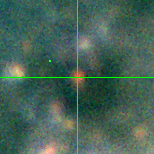M33-013400.22 (J013400.22+304047.5)

## Target Notes### Classification: Fe_emission

Likely to be a star cluster with a luminous massive star in it. Two stars approximately 2 magnitudes fainter (J013400.23+304045.1 and J013400.24+304045.0) within 2.5. arcseconds. The PSF fit for this target in V images is terrible because it sits on a discontinuity in the background (which is brighter in BV than RI). It is clearly a very red object. Flag all B and V as "B", RI as "b."

## Plots of Brightness and Color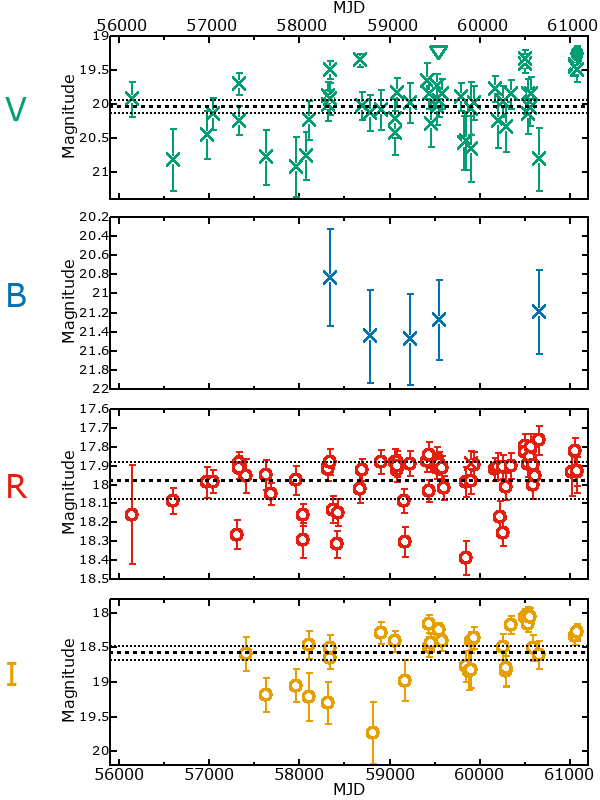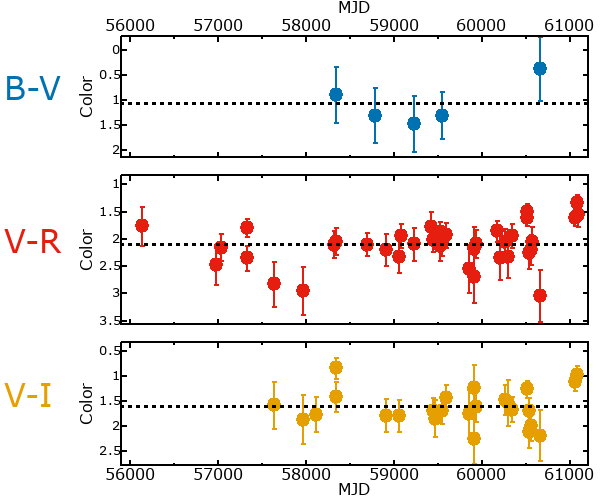## Variability

From the data available in this survey, it is not possible to tell with certainty if this star is variable.

### Correlation factors between bands

If the brightness variation is true variability then there should be a correlation between brightness changes recorded in different filter bands. The following table are the R-squared linear correlation factors calculated for the pairs of bands. The number in parentheses next to the R-squared value is the number of elements used to calculate the correlation factor. The number in square brackets next to the band is the range of magnitudes measured.

V [0.00 mag]B [1.48 mag]R [0.55 mag]
B [0.00 mag]0.91 (3)
R [0.55 mag]0.06 (14)-.-- (2)
I [1.61 mag]0.79 (7)-.-- (1) 0.09 (8)

### Magnitude-Magnitude Plots

The plots below are provided to check the values in the table above and also to show any non-linear correlations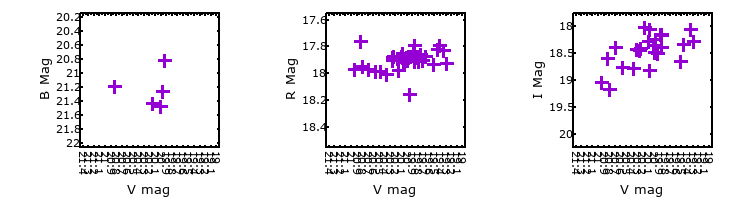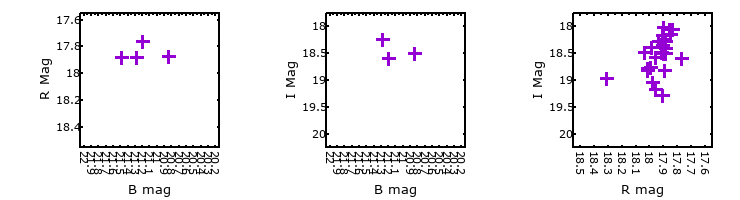### I & R Statistics

Welch & Stetson (1993, AJ, 105, 1813) present a method for varible star detection in CCD images from automated surveys. Data from two bands are compared and two statistics are calculated: R and I.

The I-statistic has an expectation value of zero for stars that are NOT variable. A larger non-zero I-value indicates a higher likelihood that the star is variable. The R statistic indicates how the color of the star changes as the variable grows brighter with R = 1.0 being no change in color.

R and I values have only been calculated if there are more than four (4) matched measurements of brightness in each band.
V
(I|R)
B
(I|R)
R
(I|R)
B
(I|R)
--- | ---
R
(I|R)
0.30 | 1.22--- | ---
I
(I|R)
1.14 | 0.63--- | --- 1.20 | 0.84